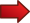The Commutative Property of Addition is one of the crucial assumptions made on Mathematics, which you probably take for granted and use all the time without knowing.

The idea of commutativity revolves around the order of an operation. The question is, do I have that

$\large a + b = b + a$

for any number $$a$$ and $$b$$? For you that may be a silly question. Like "what do you mean, of course". But commutativity does not hold for ALL operations. But it happens to be true for the common addition of numbers.Is there any proof to the commutativity of addition? Technically no, because it is rather an axiom for the real numbers as an algebraic field.Yet, but understanding how addition operates, it is easy to AGREE that commutativity makes sense, and hence, we embrace the axiom.

For example, it makes all the sense of the world to think that $$3 + 4$$ is the same as $$4 + 3$$. Why is the that?? Because of the way we conduct the addition in our minds: it is like the count 3 (say, using fingers) and then we count 4.

So we reason that we would in the end count the same amount of fingers, even if we counted 4 first and 3 second.

That is a good way of seeing it. And the take home concept out of this is that commutativity is NOT granted, and some operations will have it and others will not have it.

## Other operations that have commutativity

Is commutativity common? Yes, pretty much. But not all operations have it. Even the common ones. For example, the multiplications of numbers is commutative. This, we have that

$\large a\cdot b = b \cdot a$

for all real numbers $$a$$ and $$b$$. Nice, so that means that commutativity holds for all common operations? Not all. For example, neither the subtraction nor division of numbers are commutative. Indeed, in general

$\large a - b = \not b - a$

and the equality only holds when $$a = b$$. So for example, $$3 - 1 = 2$$ and $$1 - 3 = -2$$ are not equal. So, the subtraction of numbers is not commutative. Surprised? Well, now you know it.

Also, for the division we have that in general

$\large a / b = \not b / a$

and the equality only holds when $$a = b$$. So for example, $$6 / 3 = 2$$ and $$3 / 6 = 1/2$$ are not equal. So, the division of numbers is not commutative.

### EXAMPLE 1

Consider the following operation between real numbers $$a$$ and $$b$$:

$\large a \odot b = a\cdot b + a + b$

Is this operation commutative?

Since the addition and multiplication of real numbers is commutative, we have that

$\large a \odot b = a\cdot b + a + b = b \cdot a + b + a = b \odot a$

which implies that the operation $$\odot$$ is commutative.

### EXAMPLE 2

Now Consider the following operation between real numbers $$a$$ and $$b$$:

$\large a \odot b = a\cdot b + a + 2b$

Is this operation commutative?

Notice that

$\large a \odot b = a\cdot b + a + 2b$ $\large b \odot a = b\cdot a + b + 2a$

so then

$\large a \odot b - b \odot a = a\cdot b + a + 2b - (b\cdot a + b + 2a)$ $\large = a\cdot b + a + 2b - b\cdot a - b - 2a$ $\large = a\cdot b + a + 2b - a\cdot b - b - 2a$ $\large = a + 2b - b - 2a$ $\large = b - a$

which is not zero in general. Hence, this implies that the operation $$\odot$$ is now NOT commutative.

So, commutativity seems to be very obvious for the addition of numbers, and also for the multiplication of number. But, does it hold for all operations we can think of? Quick answer: Absolutely not.

We don't need to go too far to find examples of operations that are not commutative. For example, let us consider the multiplication of matrices. You may be surprised about it, but the multiplication of matrices is NOT commutative.

In other words, you can have matrices $$A$$ and $$B$$ for which $$A \cdot B = \not B \cdot A$$. Don't believe it? Check it out: Consider

$\large A = \left[\begin{matrix} 2 & 1 \\ -1 & 2 \end{matrix}\right] , B = \left[\begin{matrix} 1 & 1 \\ 1 & 2 \end{matrix}\right]$

Then in this case we have that

$\large A \cdot B = \left[\begin{matrix} 2 & 1 \\ -1 & 2 \end{matrix}\right] \cdot \left[\begin{matrix} 1 & 1 \\ 1 & 2 \end{matrix}\right] = \left[\begin{matrix} 3 & 4 \\ 1 &3 \end{matrix}\right]$

and

$\large B \cdot A = \left[\begin{matrix} 1 & 1 \\ 1 & 2 \end{matrix}\right] \cdot \left[\begin{matrix} 2 & 1 \\ -1 & 2 \end{matrix}\right] = \left[\begin{matrix} 1 & 3 \\ 0 & 5 \end{matrix}\right]$

which goes to show that it is NOT true in general that $$A \cdot B = B \cdot A$$.

You can read more about the commutativity property and also about the associative property . These two properties are the cornerstone foundation of the structure for real numbers.